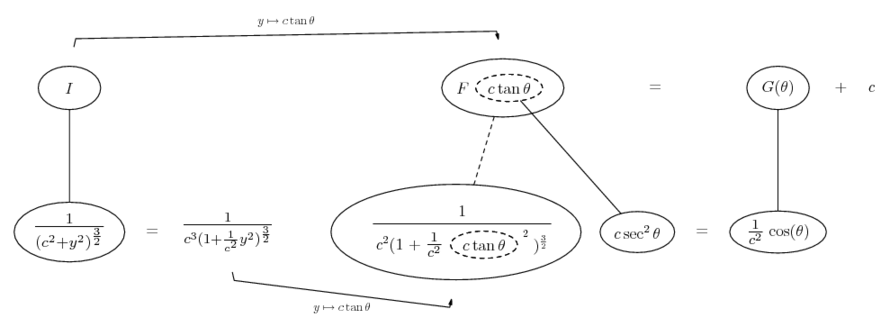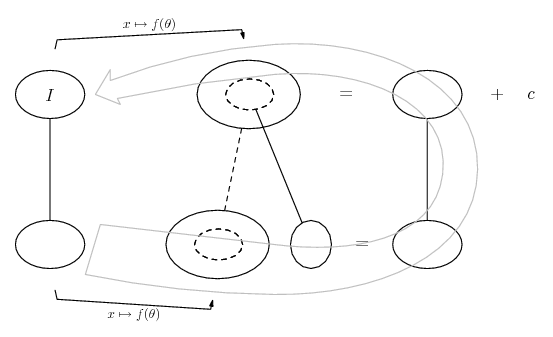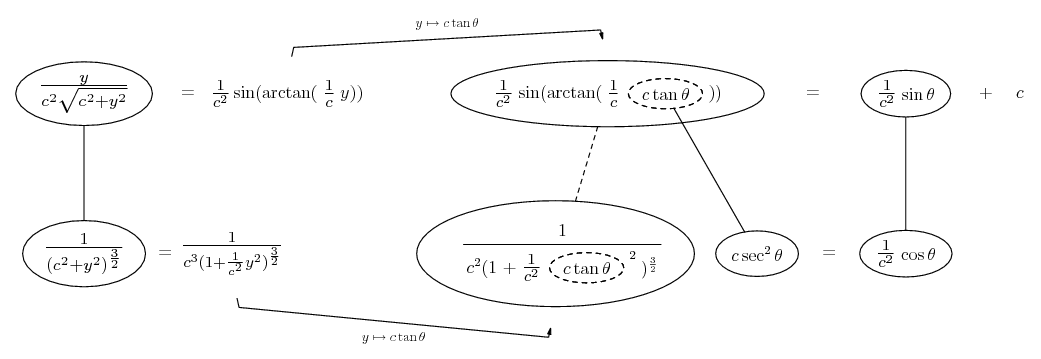# Thread: How to integrate 1/(c^2+y^2)^(3/2)?

1. ## How to integrate 1/(c^2+y^2)^(3/2)?

How do you integrate this?
Thanks!

$\displaystyle integral[1/(c^2+y^2)^(3/2)]$

ie the bottom is to the power of 3/2

2. Assuming it's with respect to y and c is constant, you can substitute

y = c tan u...

3.Originally Posted by dillonmhudsonHow do you integrate this?
Thanks!

$\displaystyle integral[1/(c^2+y^2)^(3/2)]$

ie the bottom is to the power of 3/2
substitute $\displaystyle y=c \,\ tan(\theta)$.

4. oh ok. Is there an easier way? we haven't learned the derivative of tan(/theta) yet. I'm assuming you mean do a u-substitution?

5. Just in case a picture helps...... where... is the chain rule. Straight continuous lines differentiate downwards (integrate up) with respect to the main variable (y on the left, theta after substituting), and the straight dashed line similarly but with respect to the dashed balloon expression (the inner function of the composite which is subject to the chain rule).

And I've used theta instead of u because that's usual (despite my earlier choice of u!) when you use a trig substitution to view the original function as though it were the OUTER part of the result of a chain rule differentiation. Hope the pic helps to get a handle on the logic. I'm sure others will lend further (and more conventional) advice...

The general drift is...And the rest...

Spoiler:Cheers
__________________________________________

Don't integrate - balloontegrate!

Balloon Calculus; standard integrals, derivatives and methods

Balloon Calculus Drawing with LaTeX and Asymptote!

6.Originally Posted by dillonmhudsonoh ok. Is there an easier way? we haven't learned the derivative of tan(/theta) yet. I'm assuming you mean do a u-substitution?
you have not yet learned the derivative of the tangent function, but you are finding integrals that require a trig substitution?7.Originally Posted by dillonmhudsonoh ok. Is there an easier way? we haven't learned the derivative of tan(/theta) yet. I'm assuming you mean do a u-substitution?
You do not know the derivative of the tangent function ?!
I think you mean its integral not its derivative. Right?

#### Search Tags

1 or c2, integrate, y23 or 2